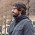Thursday, March 24, 2011

Textbook Question

[cross-posted at Choice & Inference]

Quick question: I’m excited to be teaching a course on “Reasoning and Rational Decision Making” this fall. The department’s brief course description is as follows:
Analyzing and evaluating arguments, basic logical framework, Aristotelian logic and beginning logic of sentences, fallacies, fundamentals of probability, decision theory, and game theory.
There are no prerequisites, and students taking this course are typically getting their first glimpse at logic. Clearly, expecting students to develop a thorough understanding of, and facility with, Aristotelian logic, propositional logic, probability theory, decision theory, and game theory in a course like this would be to expect too much. Thus, my larger goal for this course will be to give them a very basic understanding of the workings and objectives of basic formal logic, probability theory, and decision theory. I’ll try to spark their interests in these formal theories by showing how they can be applied to the study of human reasoning and by exploiting some fallacies, paradoxes, and the like that arise when we apply them in this way. If I have students who want to take upper-level courses in any of these formal areas as a result of my course, I’ll be very happy.

So that tells you a bit about the course and how I want to approach it. Now the question: What textbook(s) might be appropriate for a course like this? It would be ideal if there was a text that covered the basics of each of these formal theories (at a very introductory level) and does some philosophy of logic too — discussing the relation and application of the theories to human reasoning. I like Hacking’s intro to probability and inductive logic, but I would like something that spends more time on basic deductive logic … and I’d like more ideas anyway. What do you all think?

1.Richard Feldman's Reason and Argument is a wonderful text that practically teaches itself, though you would need to supplement it with probability and decision theory stuff. Feldman treats critical thinking as applied epistemology and the result is, I believe, very successful.

2.Complementing Chris's comment above, I was going to suggest that Hacking's Introduction to Probability and Inductive Logic would work well alongside a good basic formal/informal logic book. It includes very basic decision theory.

3.I agree: Hacking's book is good. I just received a desk copy of Steinhart's More Precisely, which has 1 chapter dedicated to probability theory and one dedicated to decision theory. I'll leave it in my box at Pitt for you, Jonah.

4.Thanks all! Let me know if you have any other ideas, and I'll be looking these texts over in the meantime.

5.I would use (parts of) Resnik's Choices for the decision theory section

6.Brian Skyrms' Choice and Chance.